Name:    Chapter 1 Pre Test

Multiple Choice
Identify the choice that best completes the statement or answers the question.

1.

Determine which of the numbers in the set below are integers.a.b.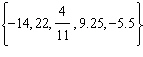c.d.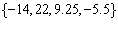e.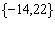2.

Find the sum.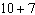a. –3 b. 70 c. –17 d. 3 e. 17

3.

Find the difference.a. 8 b. 9 c. –16 d. –8 e. 16

4.

Find the difference.a. 0 b. 14 c. –28 d. –14 e. 28

5.

What number must be added to 38 to obtain –7?
 a. 31 b. –31 c. –45 d. 45 e. 76

6.

Find the product.a.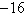b. 16 c.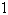d.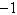e.7.

Find the product.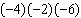a. –48 b. 192 c. 48 d. –12 e. –192

8.

Write the prime factorization of the number 432.
 a.b.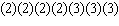c.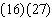d.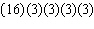e.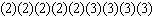9.

Find the volume of the rectangular solid.10 in. 8 in.4 in.
 a.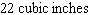b.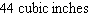c.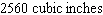d.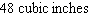e.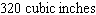10.

An integer n is divided by 3 and the quotient is an even integer. What does this tell you about n? Give an example.
 a. n is a multiple of 6,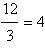b. n is a multiple of 12,c. n is a multiple of 21,d. n is a multiple of 9,e. n is a multiple of 15,11.

The following figure is divided into regions of equal area. Write a fraction that represents the shaded portion of the figure. Then write the fraction in simplest form.a.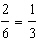b.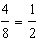c.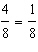d.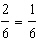e.12.

Add the fractions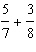and write the result in simplest form.
 a.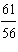b.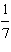c.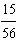d.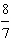e.13.

Evaluate the expressionand write the result in simplest form.
 a.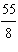b.c.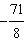d.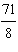e.14.

Find the reciprocal of the number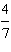.
 a.b.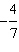c.d. –3 e.15.

Divide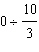if possible, and write the result in simplest form.
 a.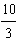b.c.d.e. not possible

16.

Evaluate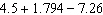and round the answer to two decimal places.
 a. –0.37 b. 5.18 c. –4.14 d. –4.15 e. –0.97

17.

Rewrite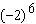as a product.
 a.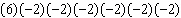b.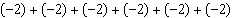c.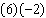d.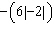e.18.

Evaluate the expression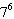.
 a.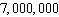b.c.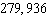d.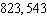e.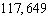19.

Evaluate the expression, if possible.
 a.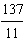b.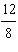c.d.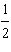e. not possible

20.

Identify the property of real numbers illustrated by the statement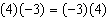.
 a. Commutative Property of Multiplication b. Commutative Property of Addition c. Distributive Property d. Associative Property of Addition e. Associative Property of Multiplication